# Wirebond Impedance and Attenuation

This page accompanies our new page on wirebonding. Some of material below was contributed by the "official" Microwaves101 Professor, thanks Dude!

### Wirebond philosophical note 1

In the coming years we are all going to see the chasm between 100 GHz and 1 THz fill in with some incredibly interesting components, modules and systems. Over recent past, many assembly houses have held on to the belief that they can always tease another decade of RF performance out of wirebonds, if they somehow make them shorter, straighter, and maybe even squirt some dielectric goop under them to add the necessary capacitance to make them into matched transmission lines. Oops, did we just mock your trade secret? How awkward... Obviously, wirebonding is attractive from a rework point of view, but recent papers and presentations at IEEE 2012 CSICS have shown people trying to use wirebonds up to 240 GHz. We have some advice: figure out another way to access your thousand-dollar amplifier. Here's two thoughts: integrated E-plane probes to waveguide, or bumps.

### Wirebond Philosophical note 2

There is a tendency on the part of microwave engineers to jump right in to a problem using 3D EM analysis. It doesn't take much thought to develop a 3D model and shoot the breeze with that hot administrator while your dual-core processor does the heavy lifting. But did you actually learn what the extent of the problem is? EM analysis has become the porn of microwave industry. Masturbative EM activities drive up the cost of doing business, as your employer needs to provide expensive licenses for this activity. Does the average microwave engineer recognize the difference between mismatch loss, radiation loss and metal loss, and what can and can't be done to control each one? Probably not, but that's why we're here to help.

Suppose your business assigned you with the task of figuring out at what frequency to stop using wirebonds. You have less skin in the game than the guy who runs the assembly room who is going to fight you over this, but that is something you will have to deal with later after you gather the facts. You should attack any fundamental microwave engineering problem this way:

4. Understand it: analyze it using algebraic equations in Excel or other programs to determine what you are up against.
5. Perform linear analysis of a nominal case using a tool such as ADS or MWO. Develop a potential solution.
6. Use Monte Carlo techniques in the linear analyzer to quantify how much trouble you can get into.
7. Use EM analysis and confirm that the linear model is behaving correctly.

Below, we will try to get you past step 4. It would be great to take this to step 7 eventually...

### Wirebond inductance

By now, we probably all know the wirebond inductance rule of thumb:

#### Wirebond inductance rule of thumb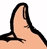A good rule of thumb is that for one-mil wire bonds (25 micron diameter), length in millimeters is nearly equal to inductance in nano-henries.

Let's take this just a step further, and use an equation for wire inductance:

The formula for bond wire inductance looks similar to that of airbridge inductance:

Lw (nH) = 5.08x10-3 * L * (ln(4*L/D) - 1)

Note that for this equation, L and D are in mils (thousandths of an inch). If you plug in 1mm length and 1 mil diameter into that equation you get 0.81 nH, which goes to show you how much you should trust a rule of thumb (Rule of thumb for rules of thumb: if it does not provide an answer that is within +/- 20% of reality, it is not very useful...) Wirebond inductance is roughly proportional to the length, but there's also a factor that accounts for the shape (length over diameter) of the wire. That may come from the fact that there are two contributors to the inductance of a wire (as opposed to a coil): one depends on the area of the loop, and the other comes from the skin effect, and it's a surface reactance that's equal to the surface resistance. The second term is pretty small compare with the first one.

The formula for bond wire inductance, plus another one that accounts for the ground plane can be found in "Computer-Aided Design of Microwave Circuits", by K.C. Gupta, Ramesh Garg and Rakesh Chadha, Artech House 1981, page 212. That book also has the same formula for inductance of a ribbon. You can find (and order) this book and many others from our microwave books page.

K.C. Gupta's book also has a correction for ribbon inductance in the presence of a ground plane. It shows the inductance of the ribbon goes down from its free-space value when a ground plane is nearby, and continues to decrease as the ground plane is brought nearer.

Gupta's book mentions the inductance of ribbon strips going down as frequency increases, to a point. This happens because of the part of the inductance that comes from the surface reactance. That reactance goes up only as the square root of frequency, which means the surface inductance is going down with the square root of frequency. At some frequency that term becomes insignificant and the other term takes over. He refers to "Calculation of Inductance of Finite-Length Strips and Its Variations with Frequency", IEEE MTT, Vol. MTT-21, 1973.

### Wirebond inductive reactance

So what does knowing the inductance of a wire tell us? Nothing, until we convert it to reactance. You could use our reactance calculator to do this, but we have saved you the trouble by plotting it below. This is for a one-millimeter long wirebond, which is typical in modules up to X-band. down at 10 GHz, you have 51 ohms of reactance, in a 50 ohm system, which is considerable, but not a killer. We will describe how to deal with this in the future. At 100 GHz, you have 510 ohms reactance, which is a train wreck.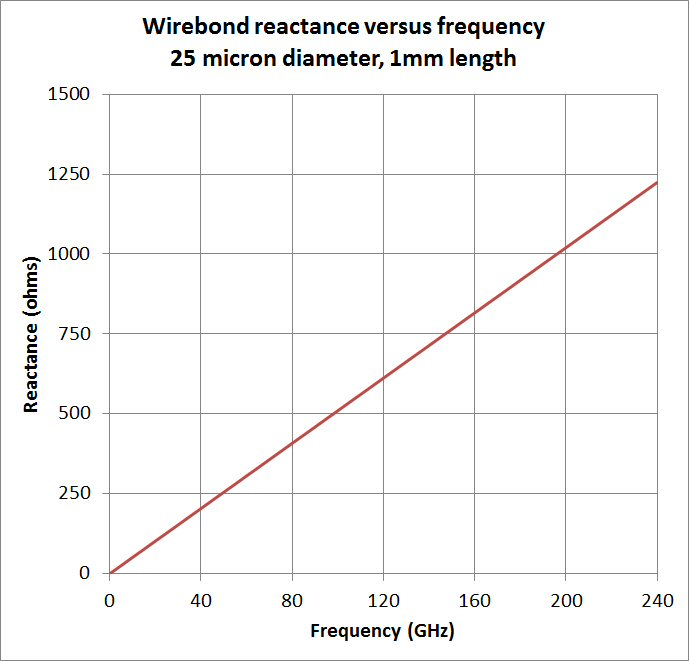Let's put out a second rule of thumb:

#### Wirebond reactance rule of thumbAnother rule of thumb is one-mil (25 micron) wire that is 1mm (40 mils) long will present about 50 ohms of reactance at 10 GHz. If you can remember this, you can scale it in your head, unless you have had a stroke and lost an entire frontal lobe.

Before we get into counteracting all that reactance, let's point out two ways to reduce it:

1. Use ribbon instead of wirebonds. This is a dual-edged sword, as ribbon bonds typically are wedge bonded instead of ball bonded, so they are straighter and shorter than wirebonds. Also, the cross-sectional area of a 1x2 mil (25x50 micron) ribbon is more than double that of a 1 mil (25 micron) wire) which reduces inductance.
2. Used two wirebonds. This solution is often used at X-band, but for MMICs about 20 GHz, it is not practical to provide an RF bond pad that can accommodate two wires because the bond pad capacitance has to be absorbed into the transition design. So all those new "E-band" MMICs have bond pads that can handle a single ribbon or wire. It should be noted that because of mutual inductance, the net inductance of two wires in parallel will not be a 50% reduction in inductance compared to a single wire, but perhaps 30-40%.

### Wirebond resistance

For wirebond resistance, we developed our own formula, based on the more accurate attenuation of coax formula we have presented here since 2006. At microwave frequencies where skin depth is less than diameter, you get one relationship where resistance increases as square root of frequency. At low frequency, resistance is nearly constant. The graph below correctly shows both effects. Note that in real life, we may be underestimating the resistance, as this calculation assumed that E-fields are all uniform around the wire; there will be some crowding on the side that is closest to a ground plane. nevertheless, this quick analysis shows 1.25 ohms at 100 GHz. We used 4.1E7 mhos/m for gold conductivity. We had to plot on log scale, otherwise this subtle effect is nearly invisible.

If you are patient we will insert the equations we used very soon, so check back! We won't propose and rules of thumb here, because it is too much to expect that anyone can scale something by square-root of frequency....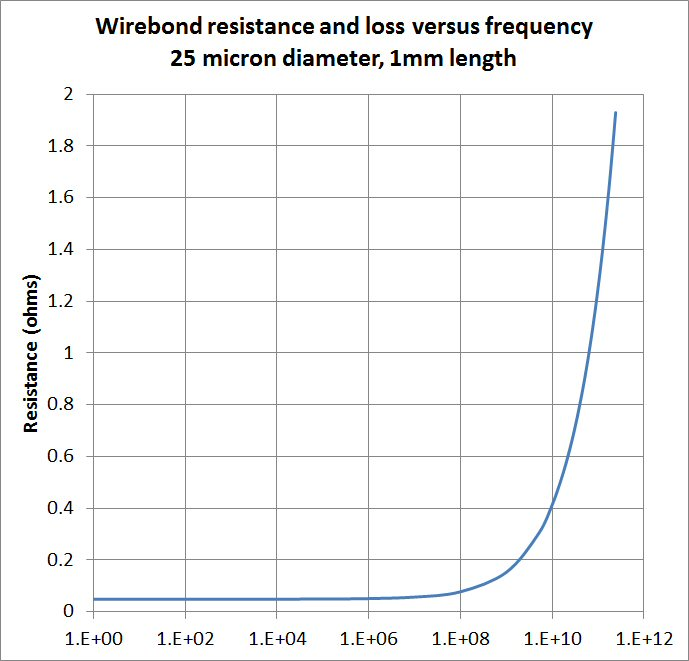### Wirebond impedance

Now let's look at them both together, on linear X-axis scale. Although we've predecided that a wirebond is a pure inductor, there is a small capacitive term that we are ignoring. Reactance scales proportionately with frequency, while resistance goes as square-root frequency. Here's a critical thinking exercise: which one should you worry about more at 240 GHz?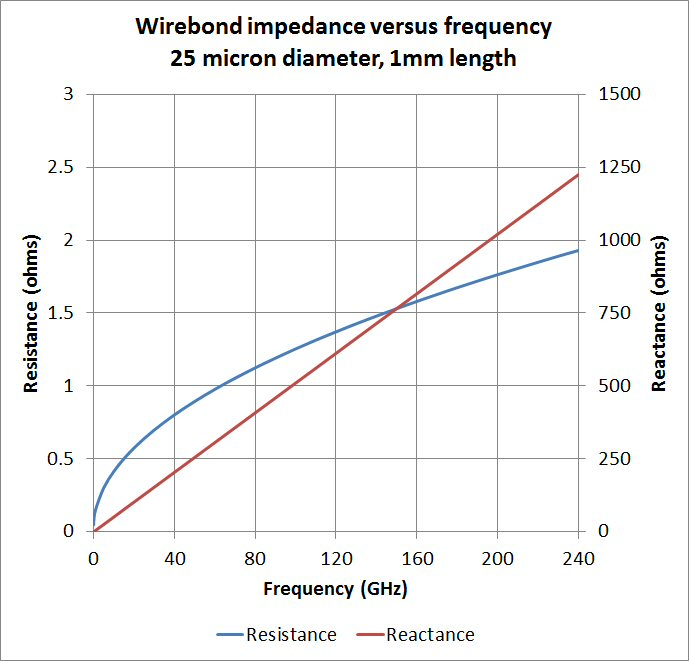### Wirebond attenuation

Last, let's look at the attenuation due to that real resistance. Remember, this is for a 1mm long wirebond, and millimeter-wave frequencies you will need to have much shorter wires. Let's project that wirebonds will never be shorter than 10 mil (250um)s, and therefore, take 1/4 of the values in the plot below to be the ultimate limit. It doesn't look all that bad, you should get less than 0.05 dB loss all the way to 240 GHz, based on this simple analysis.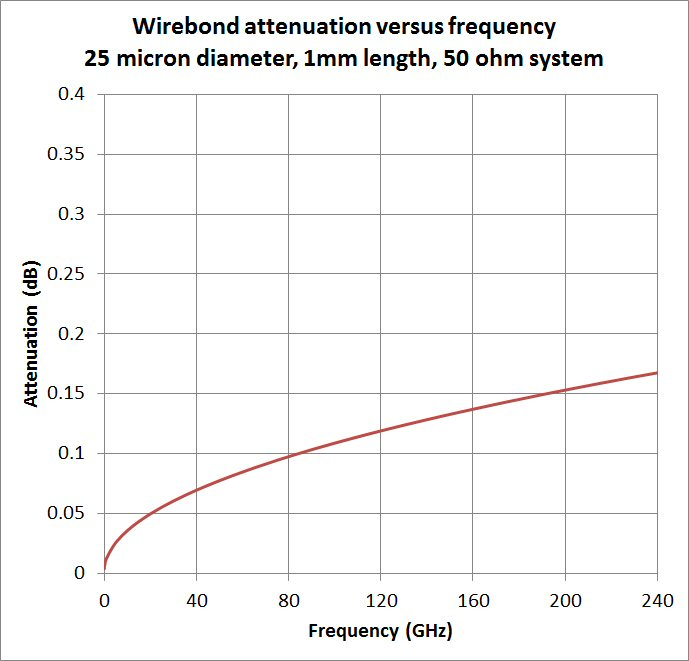But wait, there's more.... remember when said that this problem should be solved using lumped elements before you dive into EM analysis? There is a second attenuation term that is going to be important at millimeter-wave frequencies: radiation. At least now we have an idea about how much loss there is due to metal, and can therefore separate the two terms.

So what is the ultimate high-frequency limitation of wirebonds? It appears (so far) to be inductive reactance, not resistance. Remember that reactance scales proportionately with frequency, while resistance goes as square-root frequency.

But we haven't really encompassed the entire problem here. The next step it to develop a broadband matching network, then look at what happens in a Monte Carlo environment where there are real tolerances on wire length and placement.

Author : Unknown Editor